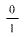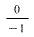# Problem: Using general tendencies, predict the most likely mode of decay of each of the following radioactive isotopes.( α decay , β decay, e emission, e capture)1. iodine-1372. sodium - 203. thorium- 2324. mercury- 1905. potassium- 47

###### FREE Expert Solution
86% (212 ratings)
###### FREE Expert Solution

We’re being asked to predict the most likely mode of decay for each of the following radioactive isotopes

Recall that the stability of an isotope depends on the ratio of neutrons to protons (N/Z). This means for stable isotopes with:

• Z ≤ 20; the N/Z ratio should be equal to 1.0

• 20 < Z ≤ 40; the N/Z ratio should be equal to 1.25

• 40 < Z ≤ 80; the N/Z ratio should be equal to 1.50

• Z < 83; the stable nuclide does not exist

The N/Z values for stable isotopes form the valley or band of stability:This means for isotopes:

• above the valley of stability: there too many neutrons in the isotope

Mode of decay: Either beta decay (0–1β) or neutron emission (10n; not in the choices)

• below the valley of stability: there too many protons in the isotope

Mode of decay: Either positron emission (01e) or electron capture (0–1e)

• with atomic mass ≥ 200 amu: there too many protons and neutrons in the isotope

Mode of decay: alpha decay (42α)

86% (212 ratings)###### Problem Details

Using general tendencies, predict the most likely mode of decay of each of the following radioactive isotopes.

( α decay , β decay,e emission,e capture)

1. iodine-137

2. sodium - 20

3. thorium- 232

4. mercury- 190

5. potassium- 47

What scientific concept do you need to know in order to solve this problem?

Our tutors have indicated that to solve this problem you will need to apply the Band of Stability concept. You can view video lessons to learn Band of Stability. Or if you need more Band of Stability practice, you can also practice Band of Stability practice problems.

What is the difficulty of this problem?

Our tutors rated the difficulty ofUsing general tendencies, predict the most likely mode of de...as high difficulty.

How long does this problem take to solve?

Our expert Chemistry tutor, Dasha took 10 minutes and 51 seconds to solve this problem. You can follow their steps in the video explanation above.

What professor is this problem relevant for?

Based on our data, we think this problem is relevant for Professor McCurdy's class at IOWA.